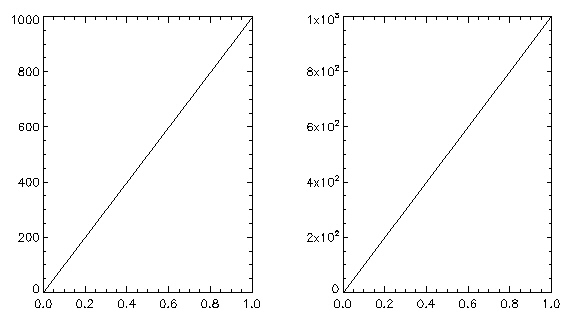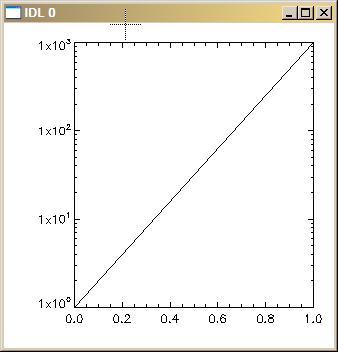# Forcing Exponential Axis Annotation

QUESTION: It seems that IDL has some kind of internal algorithm that sometimes selects exponential axis tick labeling and sometime selects number axis tick labeling. Can I force it to use exponential tick labeling all of the time?

ANSWER: You can create tick labels any way you like by using a special tick labeling function. The function is called by specifying the name of the function using the [XYZ]TickFormat keyword to a graphics command.

Here is a tick label function for forcing exponential axis labeling written by Stein Vidar Hagfors Haugan. Notice that the function is defined with three positional parameters. These are required for a tick formatting function.

```   FUNCTION Exponent, axis, index, number

; A special case.
IF number EQ 0 THEN RETURN, '0'

; Assuming multiples of 10 with format.
ex = String(number, Format='(e8.0)')
pt = StrPos(ex, '.')

first = StrMid(ex, 0, pt)
sign = StrMid(ex, pt+2, 1)
thisExponent = StrMid(ex, pt+3)

; Shave off leading zero in exponent
WHILE StrMid(thisExponent, 0, 1) EQ '0' DO thisExponent = StrMid(thisExponent, 1)

; Fix for sign and missing zero problem.
IF (Long(thisExponent) EQ 0) THEN BEGIN
sign = ''
thisExponent = '0'
ENDIF

; Make the exponent a superscript.
IF sign EQ '-' THEN BEGIN
RETURN, first + 'x10!U' + sign + thisExponent + '!N'
ENDIF ELSE BEGIN
RETURN, first + 'x10!U' + thisExponent + '!N'
ENDELSE

END
```

To see how it works, first try this command:

```  IDL> Plot, [1, 1e3]
```

And then try it with the tick formatting function, like this:

```  IDL> Plot, [1, 1e3], YTickFormat='exponent'
```

Here is an example that shows how the Y axis labels should appear after typing the two commands above.Example plot with and without EXPONENT function tick formatting.

This function can even be used with logarithmic axes, like this:

```  IDL> Plot, [1,1e3], YTickFormat='exponent', YLog=1
```The EXPONENT function can also be used with log plots.

Paul van Delst has written a similar tick formatting function, that he describes in this IDL Newsgroup article.[Return to IDL Programming Tips]